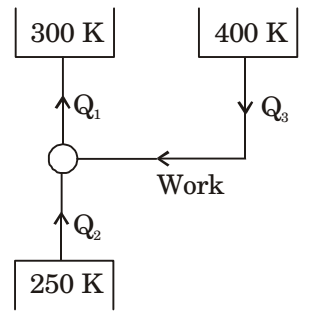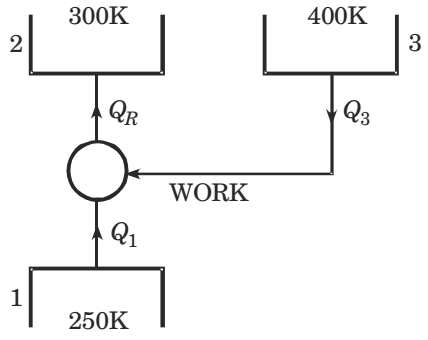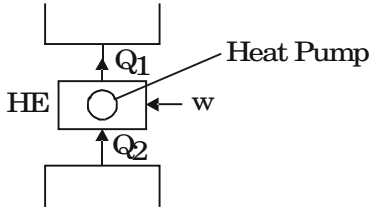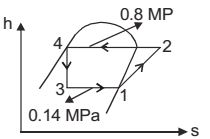## Refrigeration and Air-conditioning Miscellaneous

#### Refrigeration and Air-conditioning

1. A vapour absorption refrigeration system is a heat pump with three thermal reservoirs as shown in the figure. A refrigeration effect of 100 W is required at 250 K when the heat source available is at 400 K. Heat rejection occurs at 300 K. The minimum value of heat1. From question, since refrigeration effect of 100 W is required

 So, 100 = 250 Work obtained 300 – 250

 Work obtained = = 100 × 50 = 20 W 250Now for this amount of work, heat is absorbed from reservoir 3 and rejected to sink 2.
 ∴ η = W = 1 - 300 = 1 Q3 400 4

⇒ Q3 = 4W = 4 × 20 W = 80 W

##### Correct Option: C

From question, since refrigeration effect of 100 W is required

 So, 100 = 250 Work obtained 300 – 250

 Work obtained = = 100 × 50 = 20 W 250Now for this amount of work, heat is absorbed from reservoir 3 and rejected to sink 2.
 ∴ η = W = 1 - 300 = 1 Q3 400 4

⇒ Q3 = 4W = 4 × 20 W = 80 W

1. An industrial heat pump operates between the temperatures of 27°C and -13°C. The rates of heat addition and heat rejection are 750 W and 1000 W, respectively. The COP for the heat pump is

1. Heat addition, Q2 = 750 W
Heat rejected, Q1 = 1000 W

 [C.O.P]H.P = Q1 = Q1 W Q1 - Q2

 = 1000 = 1000 = 4 1000 - 750 250

T1 = 273 + 27º = 300ºKT2 = 273 – 13 = 260K
 [C.O.P]H.P = Q1 Q1 - Q2

for actual heat pump
 = 1000 = 1000 = 4 1000 - 750 250

##### Correct Option: C

Heat addition, Q2 = 750 W
Heat rejected, Q1 = 1000 W

 [C.O.P]H.P = Q1 = Q1 W Q1 - Q2

 = 1000 = 1000 = 4 1000 - 750 250

T1 = 273 + 27º = 300ºKT2 = 273 – 13 = 260K
 [C.O.P]H.P = Q1 Q1 - Q2

for actual heat pump
 = 1000 = 1000 = 4 1000 - 750 250

1. List-I
A. Liquid to suction heat exchange
C. Normal shock
D. Ammonia-water
List-II
1. Vapour absorption refrigeration
2. Vapour compression refrigeration
3. Diesel cycle
4. Otto cycle
5. Converging nozzle
6. Converging diverging nozzle

1. A – 2, B – 4, C – 6, D – 1

##### Correct Option: A

A – 2, B – 4, C – 6, D – 1

1. Clearance volume of a reciprocating compressor is 100 ml, and the volume of the cylinder at bottom dead centre is 1.0 litre. The clearance ratio of the compressor is

1.  Clearance ratio = Clearance volume Swept volume

 = 100 = 1 1000 - 100 9

##### Correct Option: C

 Clearance ratio = Clearance volume Swept volume

 = 100 = 1 1000 - 100 9

1. A refrigerator uses R134a as its refrigerant and operates on a ideal vapour-compression refrigeration cycle between 0.14 MPa and 0.8 MPa. If the mass flow rate of the refrigerant is 0.05 kg/s, the rate of heat rejection to the environment is _______ kW.
Given data:
At P = 0.14 MPa, h = 236.04 kJ/kg , s = 0.9322 kJ/kgK
At P = 0.8 MPa, h = 272.05 kJ/kg (superheated vapour)
At P = 0.8 MPa, h = 93.42 kJ/kg (saturated liquid)

1.h1 = 236.04 kJ/kg
h2 = 272.05 kJ/kg
h3 = 93.42 kJ/kg
s1 = 0.9322 kJ/kg
ṁ = 0.05 kg/s
Heat Rejection = ṁ(h2 – h3) = 0.05(272.05 – 93.42)
Heat Rejection = 8.931 kW

##### Correct Option: Ah1 = 236.04 kJ/kg
h2 = 272.05 kJ/kg
h3 = 93.42 kJ/kg
s1 = 0.9322 kJ/kg
ṁ = 0.05 kg/s
Heat Rejection = ṁ(h2 – h3) = 0.05(272.05 – 93.42)
Heat Rejection = 8.931 kW